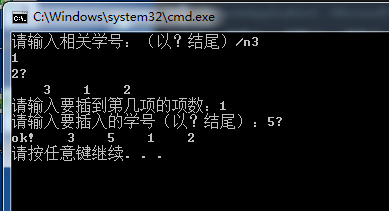c语言链表建立的问题。。。求人来解答
``````#include<stdio.h>
#include<malloc.h>
#include<stdlib.h>
typedef struct student
{int number;
struct student *next;
} NODE;
typedef struct student node;
{
int a;
char ch;
printf("请输入相关学号：（以？结尾）/n");
while(ch!='?')
scanf("%d",&a);
q->number=a;
p->next=q;
p=q;
ch=getchar();
}
p->next=NULL;
}
{int i,m; char ch; link ptr;
for(i=0;i<a;i++)
printf("请输入要插入的学号（以？结尾）：");
while(ch!='?')
{scanf("%d",&m);
ptr->number=m;
ch=getchar();
return 0;
}
}
}
printf("\n") ;
}
int main()
a=creatlist();
print(a);
printf("请输入要插到第几项的项数：");
scanf("%d",n);
printf("ok!");
print(a);
return 0;
}
``````

ps:觉得麻烦的话不用改正，告诉我为什么会出现这种情况就行了。

3个回答

1.你的main函数里的scanf少了个&
2.然后我接着看了你的creatlist函数，你这个输入必须是输入一个数按一下回车，最后输入的数还必须加上？再按回车才行`````` #include<stdio.h>
#include<malloc.h>
#include<stdlib.h>
typedef struct student
{
int number;
struct student *next;
} NODE;
typedef struct student node;
{
int a;
char ch = ' ';
printf("请输入相关学号：（以？结尾）/n");
while (ch != '?')
{
scanf("%d", &a);
q->number = a;
p->next = q;
p = q;
ch = getchar();
}
p->next = NULL;
}
{
int i, m; char ch = ' '; link ptr;
for (i = 0; i<a; i++)
printf("请输入要插入的学号（以？结尾）：");
while (ch != '?')
{
scanf("%d", &m);
ptr->number = m;
ch = getchar();
return 0;
}
}
{
{
}
printf("\n");
}
int main()
{
a = creatlist();
print(a);
printf("请输入要插到第几项的项数：");
scanf("%d", &n);
printf("ok!");
print(a);
return 0;
}
``````C语言链表的一道题目 求解答
C语言动态链表建立问题
#include<stdio.h> #include<stdlib.h> #define LEN sizeof(struct Student) int n = 0;//记录数据的个数 struct Student { int number; int score; struct Student *next; }; int main() { struct Student *creat(void); void print(struct Student *p); struct Student *p; p = creat(); print(p); return 0; } struct Student *creat(void) { struct Student *p1,*p2; struct Student *head = NULL; p1 = p2 = (struct Student *)malloc(LEN); printf("please enter Students' informations(number & score:)"); scanf("%d %d",&p1->number,&p1->score);//get while(p1->number != 0) { n += 1; if(n==1)head = p1; else p2->next = p1; p2 = p1; printf("please enter Students' informations(number &score):"); scanf("%d %d",p1->number,p1->score); p1 = (struct Student*)malloc(LEN); } p2->next = NULL; return (head); }; void print(struct Student *p) { printf("their informations as follows:\n"); while(p->number!=0) { printf("%d %d",p->number,p->score); p = p->next; } } 代码如上，运行后输入第二个数据后显示“该内存不能为written”,用的是CODEBLOCKS。卡在这一天了，求解答，万分感谢~
C语言链表文件题请看看这个有什么问题
``` #include<stdio.h> #include<string.h> #include<stdlib.h> struct stu{ int num; char name; int score; };//定义一个学生信息结构体 struct st{ int num; char name; int score; struct st *next; };//定义一个学生信息链表结点 struct st *top,*rear;//定义链表头尾指针 struct st* push (struct stu x) { struct st *p; p=(struct st*)malloc(sizeof(struct st)); p->num=x.num; strcpy(p->name,x.name); p->score=x.score; p->next=NULL; if(rear==NULL) { rear=top=p; } else{ rear->next=p; rear=p; } return p; }//将结构体元素依次放入链表尾 void sort (struct st *top) { int flag; struct st *q,*s; int temp1,temp3; char temp2; for(q=top->next;q->next!=NULL;q=q->next) { flag=0; s=q->next; if(q->num<s->num) { flag=1; temp1=q->num; q->num=s->num;s->num=temp1; temp3=q->score;q->score=s->score;s->score=temp3; strcpy(temp2,q->name);strcpy(q->name,s->name);strcpy(s->name,temp2); } if(flag==0) break; } }//按照学号从大到小的顺序进行排序节点 int main() { FILE *in,*out; in=fopen("in.txt","r"); out=fopen("out.txt","w");//打开文件 int n,m; struct stu card; scanf("%d",&n); m=n; while(m!=0) { fread(&card,sizeof(struct stu),1,in); if(card.num>0) { m--; push(card); } }//若学号符合要求则连人链表 sort(top); struct st *k; for(k=top;k!=NULL;k=k->next) { fwrite(&card,sizeof(struct stu),1,out); }//写入out文件 fclose(in); fclose(out);//关闭文件 return 0; } ``` 为啥运行后没有反应呢 out文件中没有我想要的数据
c语言链表删除节点问题
#include <stdio.h> #define NUll 0 #include <stdlib.h> struct stu { char name; int xuehao; int grade; struct stu *next; }; typedef struct stu STU; main() { STU *p,*p_start,*p2,*p_print,*p_charu,*p_charu2; int i,xuehao; //输入 for (i=0;i<3;i++) { p=(STU *)malloc(sizeof(STU)); printf("请输入学生姓名：\n"); scanf("%s",&p->name); printf("请输入学生的学号：\n"); scanf("%d",&p->xuehao); printf("请输入学生的成绩：\n"); scanf("%d",&p->grade); if (i==0) p2=p_start=p; else { p2->next=p; p2=p; if (i==2) p->next=NULL; } } // printf("%d",p_start->next->next->next); // printf("%d",p_start->next->next->xuehao); //链表的插入 p_charu=p_start; printf("请输入要删除的学号\n"); scanf("%d",&xuehao); while (1) { p_charu2=p_charu->next; if (p_charu->next->xuehao==xuehao) { // if (p_charu->next->next==NULL) // p_charu->next=NULL; else p_charu->next=p_charu->next->next; free(p_charu2); break; } if (p_charu->xuehao==xuehao) { p_start=p_charu->next; free(p_charu); break; } p_charu=p->next; } //输出 p_print=p_start; /* for (i=0;i<2;i++) { printf("学生姓名为:%s\n",p_print->name); printf("学生的学号为:%d\n",p_print->xuehao); printf("学生的分数为:%d\n",p_print->grade); if (p_print->next!=NULL) p_print=p_print->next; } */ } 这是一个删除结点并输出的程序， 加注释的输出部分没有问题，关键应该在删除结点的循环那里，但是找不见错误啊， 错误为：当删除最后一个链表也就是3时程序发生错误，停止运行。 求解
C语言链表数据输入问题

``` #include<stdio.h> #include<stdlib.h> struct node { int num; int L; struct node *next; }; typedef struct node LN; LN *Creat(int n); void print(LN *h); void Revise(LN *p); void main() { LN *h; int a; printf("Input the sum of the number:"); scanf("%d",&a); h=Creat(a); system("CLS"); Revise(h); print(h); } LN *Creat(int n) { LN *head; LN *p; LN *b; head=(LN *)malloc(sizeof(LN)); head->next=NULL; b=head; for(int i=1;i<=n;i++) { printf("Input the %d number:",i); p=(LN *)malloc(sizeof(LN)); scanf("%d",&p->num); b->next=p; b=p; p->L++; } system("CLS"); p->next=NULL; return head; } void print(LN *h) { LN *p; p=h->next; while(p) { printf("%d\n",p->num); p=p->next; } } void Revise(LN *p) { LN *a; LN *b; int n; int i=0; int data; printf("Input which node you want to insert："); scanf("%d",&n); printf("Please input the data："); scanf("%d",&data); while (i<n-1) { p=p->next; i++; } a=(LN *)malloc(sizeof(LN)); b=(LN *)malloc(sizeof(LN)); p->num=data; a=p->next; p->next=b; b->next=a; } ``` 其中在链表插入当中插入错误，插入节点是对的，但是数就是错的。
c语言链表节点删除问题求解

c语言存储链表数据问题

C语言新人关于链表的问题

c语言链表问题，希望能在10号之前得到解答

C语言线性链表建立问题

C语言：合并有序双向链表问题

【C语言-链表】请问怎么判断链表已经被销毁？
Java学习的正确打开方式

linux系列之常用运维命令整理笔录

Python十大装B语法
Python 是一种代表简单思想的语言，其语法相对简单，很容易上手。不过，如果就此小视 Python 语法的精妙和深邃，那就大错特错了。本文精心筛选了最能展现 Python 语法之精妙的十个知识点，并附上详细的实例代码。如能在实战中融会贯通、灵活使用，必将使代码更为精炼、高效，同时也会极大提升代码B格，使之看上去更老练，读起来更优雅。

Docker 技术发展为微服务落地提供了更加便利的环境，使用 Docker 部署 Spring Boot 其实非常简单，这篇文章我们就来简单学习下。首先构建一个简单的 S...

SQL-小白最佳入门sql查询一

redis分布式锁，面试官请随便问，我都会

Nginx 原理和架构
Nginx 是一个免费的，开源的，高性能的 HTTP 服务器和反向代理，以及 IMAP / POP3 代理服务器。Nginx 以其高性能，稳定性，丰富的功能，简单的配置和低资源消耗而闻名。 Nginx 的整体架构 Nginx 里有一个 master 进程和多个 worker 进程。master 进程并不处理网络请求，主要负责调度工作进程：加载配置、启动工作进程及非停升级。worker 进程负责处...
“狗屁不通文章生成器”登顶GitHub热榜，分分钟写出万字形式主义大作

《程序人生》系列-这个程序员只用了20行代码就拿了冠军

11月8日，由中国信息通信研究院、中国通信标准化协会、中国互联网协会、可信区块链推进计划联合主办，科技行者协办的2019可信区块链峰会将在北京悠唐皇冠假日酒店开幕。 　　区块链技术被认为是继蒸汽机、电力、互联网之后，下一代颠覆性的核心技术。如果说蒸汽机释放了人类的生产力，电力解决了人类基本的生活需求，互联网彻底改变了信息传递的方式，区块链作为构造信任的技术有重要的价值。 　　1...
Java世界最常用的工具类库
Apache Commons Apache Commons有很多子项目 Google Guava 参考博客

【技巧总结】位运算装逼指南

Android 9.0 init 启动流程

8年经验面试官详解 Java 面试秘诀